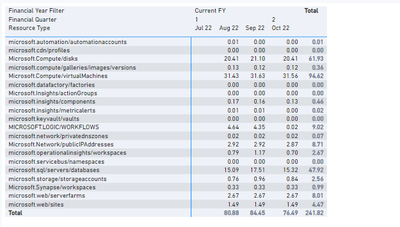cancel
Showing results for
Did you mean:Frequent Visitor

## Keep row values as sum, but change total as average in matrix

As the title implies, is it possible to change the total of a matrix into average without changing the row values (sum)? I have seen solutions where it involves modifying the column and just creating measures for column values, but the column headers should be dynamic as they are date columns and they will add on the longer the time goes. Kindly see sample data below. I know there is a way to have Monthly row totals be made into measures and show that into the table instead, but that is not really dynamic. Can anyone please help?

Thank you.1 ACCEPTED SOLUTIONCommunity Support

Hi, @noobpowerbiuser ;

You could create a measure

``````Measure =
IF (
ISINSCOPE ( 'Table'[Date].[Year] ),
SUM ( 'Table'[values] ),
AVERAGEX (
SUMMARIZE (
'Table',
[Catetory],
[Date].[Year],
[Date].[Month],
"1", SUM ( 'Table'[values] )
),

)
)
``````

The final show:Best Regards,
Community Support Team _ Yalan Wu
If this post helps, then please consider Accept it as the solution to help the other members find it more quickly.

4 REPLIES 4Community Support

Hi, @noobpowerbiuser ;

You could create a measure

``````Measure =
IF (
ISINSCOPE ( 'Table'[Date].[Year] ),
SUM ( 'Table'[values] ),
AVERAGEX (
SUMMARIZE (
'Table',
[Catetory],
[Date].[Year],
[Date].[Month],
"1", SUM ( 'Table'[values] )
),

)
)
``````

The final show:Best Regards,
Community Support Team _ Yalan Wu
If this post helps, then please consider Accept it as the solution to help the other members find it more quickly.Super User

you can use HASONEVALUE to check if you are on a Resource Type row like this.

``````Amount Measure =
IF (
HASONEVALUE ( 'Table'[Resource Type] ),
SUM ( 'Table'[Amount] ),
AVERAGEX ( VALUES ( 'Table'[Resource Type] ), CALCULATE ( SUM ('Table'[Amount] ) ) )
)``````Frequent Visitor

Sorry, this makes the column totals as average, but I want the row totals in average. Basically just switched aroundSuper User

@noobpowerbiuser , Assume you are using a measure M1 in the matrix

AverageX(Summarize(Fact, Date[FY Year], Date[Qtr], Fact[Resource Group]), [M1])